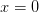# Of Rotation, LT and Acceleration

We defined the rotation in 2-D Euclidean space as X’ = R X where the matrix of rotation R is,$\left[ {\begin{array}{*{20}c}{\cos \theta } & { - \sin \theta } \\{\sin \theta } & {\cos \theta } \\\end{array}} \right]$

So, the matrix equation expands towhere$\theta$ is the angle of rotation.

Let’s try to visualize a situation where$\theta$ is a function of$x$, say$\theta(x) = x$. Imagine a sheet of paper with$x$ along one edge and$y$ along another. At$x=0$, there is no rotation. As x increases, there is more and more rotation. So the edge of the paper corresponding to the x axis becomes something like a parabola, while the y axis stays put. If you manage to do this, the sheet of paper sticks out and is pretty @#\$%in’ far from flat (as Marsellus of Pulp Fiction would’ve put it).

To see why it is not Euclidean, imagine drawing a line at$y=1$, perpendicular to the y-axis. It will soon intersect the x-axis because the x-axis is curving up. At the point where it intersects the x-axis, it will make an angle say$\phi$. Now, look at the triangle made up of the x-axis, y-axis and this line. The sum of the angles is$\pi+\phi$, violating the property that the sum should be$\pi$ for Euclidean space.

Once the space is non-flat, we have to stop applying our intuitively correct numerical or calculus techniques to it. When we apply such techniques, we are working on the coordinates. The notion of coordinates makes no sense in a non-flat geometry. The metric tensor itself becomes position dependent, which is hard to express because you lack coordinates. In other words, the whole thing becomes horribly complicated. But somebody had actually worked it all out in the 19th century. Riemann, for reasons only known to himself, figured out how to do this in a general way.

It is in this sense that space-time is curved in GR, not so much as like the popular illustrations in which you have a bowling ball on a stretched rubber sheet and marbles going around it mimicking a solar system.

This warping is exactly what happens when the LT matrix depends on time through acceleration. It is a lot harder to visualize though, because of two things: One, the metric tensor is [-1, 0 ; 0, 1], which is not the same as the identity matrix and the associated geometry that we can visualize. Two, time has a strong semantic context in our mind so that we may still think that LT matrix is constant for small durations of time so that we may still use the flat Minkowski geometry. The only right way to do it, however, is to employ a much stronger geometry, which is Riemannian, in Einstein’s opinion.

Before I sign off, I would like to highlight one aspect of Einstein’s genius in doing all this. Sure, he doesn’t need any accolade from this dissenting admirer, and this forum is hardly the place to express it. Nevertheless. Einstein’s genius lies not so much in the accuracy of his theories, but in taking a simple principle that nobody can argue with and developing it farther than anybody thought possible. For instance, in GR, he starts with the principle that acceleration is equivalent to gravity because a freely falling body experiences no gravity. Who can argue with that? Then he says acceleration results in a warped space-time, as we saw here. Weird and impossible to visualize, but logical. Then he adopts Riemannian geometry to describe the curved space-time, and voila GR!

Continued…